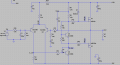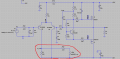# Difference in calculated and Spice result of DC bias current (Audio Amp)

#### janjan22

Joined Jun 10, 2015
14
Hi,

I'm experimenting with a schematic of an audio amp in LTspice. First I looked at the DC bias currents of the amp with the ".op directive". The results showed bias currents:

IC(Q2) = 0.929 mA
I(R2) = 0.916 mA
IB(Q4) = 0.0125 mA
IC(Q4) = 4.83 mA

This Spice result for I(R13) (base current going to Q4) is lower than my own calculations.

First I calculated the intrinsic emitter resistance of Q4:
re = Vt / IC(Q4) = 26mV/4.83mA = 5.41 ohm.

Then i drawed the schematic to determine the equivalent resistance that the collector of Q2 sees (in the red highlighted area).

The input resistance of Q4 is:

Beta(Q4) * re = 294.3 *5.41 ohm = 1.59K (I looked up the current gain in the model parameters of the BC546B, BF=294.3)
This resistance is in series with R13 --> 4.7K + 1.59K = 6.29K

The current into the base of Q4 can be calculated with the current division rule:

IB(Q4) = 800 / (800 + 6.29K) * IC(Q2) = 0.11 * 0.929 mA = 0.102 mA

As you can see this does not match with the Spice result (IB(Q4) = 0.0125 mA).
Has anyone an idea if my calculations are correct?#### Jony130

Joined Feb 17, 2009
5,445
I do not have time right now to analysis fully yore question. But from what I see you mix a DC bias currents with small-signal (AC) parameters.
re - intrinsic emitter resistance is a small-signal parameter and you cannot use it in DC calculations the way you did. re can only be used when you are doing the AC analysis (small-signal).

#### Bordodynov

Joined May 20, 2015
3,059
Part of scheme is disconnected On the second your picture. As a result this one of the transistor differential pair(vapour)s out-of-work. The Whole current goes through the first transistor. Load its file of the scheme (asc-file), Then I shall draw you correct equivalent scheme.

#### janjan22

Joined Jun 10, 2015
14
Yes, part of the schematic of the second picture is indeed disconnected. I just made it to show the total resistance that is seen from the collector of Q2. Just for graphical purpose.

#### Bordodynov

Joined May 20, 2015
3,059
As you have already written, your approach to the problem is wrong. I can draw (from your circuit) equivalent circuit, which will be true for DC and AC. I am too lazy to enter your circuit and so I asked to load your circuit. if you do not do this, I wash my hands.
Parameter model BF can not be equal to the gain of the transistor. Gain transistor is not constant but depends on the mode transistor.

Last edited:

#### dannyf

Joined Sep 13, 2015
2,197
The current into the base of Q4 can be calculated with the current division rule:

IB(Q4) = 800 / (800 + 6.29K) * IC(Q2) = 0.11 * 0.929 mA = 0.102 mA
Not really: the 6.29K figure is an ac concept.

The DC base current is indeterminant - at least not without some plotting. The right thing to do is to use a Re resistor on Q4 to explicitly set Ic -> you don't care about Q4's Ib unless it is sufficiently close to the current through that 800ohm resistor, whose value is too low for a properly designed amp.

#### Bordodynov

Joined May 20, 2015
3,059

#### janjan22

Joined Jun 10, 2015
14
Hi Bordodynov

Attached is the schematic of the amp.
About BF: what does BF represent? I thought it was the DC current gain.

@danny:
The DC base current is indeterminant - at least not without some plotting.
What do you mean with plotting? How can we determine the input resistance of a BJT for DC? And finally why is this 800 ohm value too low? Thanks

#### Attachments

• 5.5 KB Views: 2

#### Bordodynov

Joined May 20, 2015
3,059

#### Jony130

Joined Feb 17, 2009
5,445
Ib4 = Ic4 /β. And for this circuit Ic4 can be easily found and we have much greater "control" over Ic4 thanks to the global negative feedback. And Ib4 is depending on the Beta value. And as you should know the β is not constant. Beta varies with Ic, Vce, temperature. And what is worse, every single transistor will have different beta.

As for this BF in LTspcie this BF is a ideal maximum forward beta.

#### Bordodynov

Joined May 20, 2015
3,059

#### dannyf

Joined Sep 13, 2015
2,197
What do you mean with plotting? H
Oems used to provide an IC vs. Vce curve for a series Ib. You can plot out where your transistor is on that chart for its Ib.

#### dannyf

Joined Sep 13, 2015
2,197
And finally why is this 800 ohm value too low? T
The way to work out the VAs is to figure out its Ic first. In this case, it is about 30v / 5.4k or 5ma.

You will then figure out the VAs gain by setting the Re. I would pick something less than 110ohm. Let's say we pick a 47ohm resistor here. The voltage drop on it is about 250mv. So the voltage drop over the resistor on the long tail is 0.7v + 0.25v or 1v. It's current is 1ma thus that 800ohm resistor is really a 1k resistor. At this point you may want to verify that q4 has sufficient gain so that not draw too much current away from the 1k resistor. This approach allows the output to sit at roughly ground even if it is open loop.

That's the general design process.